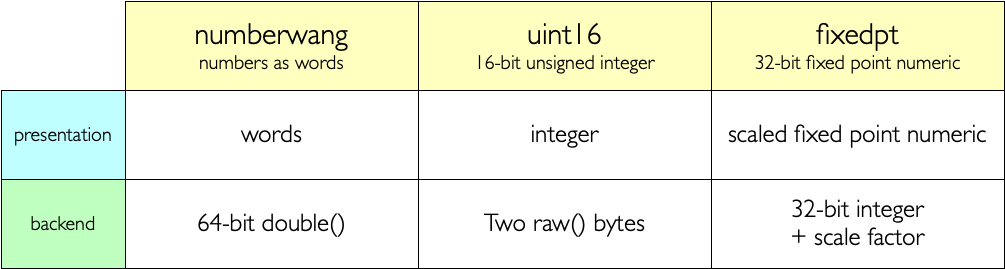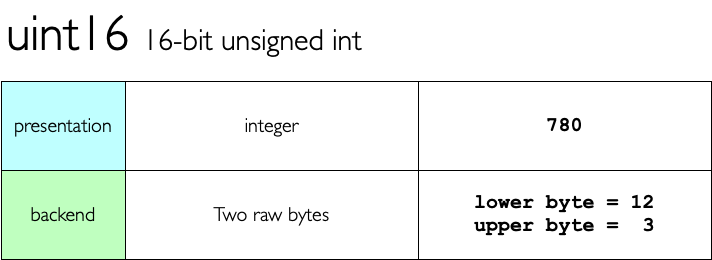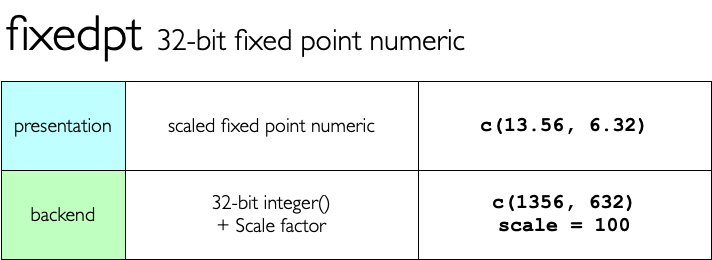# typhoid - non-standard numeric datatypes`typhoid` provides implementations of some non-standard datatypes using `{vctrs}`## What’s in the box

• `numberwang(x)` for creating numeric values which present themselves as words.
• `uint16(x)` for creating 16-bit unsigned integers, where each value is stored as a pair of raw bytes.
• `fixedpt(x, decimals = 3)` for creating 32-bit fixed point numeric values.

Each of the types supports `as_<type>()` and `is_<type>()`, as well as standard arithmetic operations (`+`, `*` etc) and mathematical functions e.g. `abs()`

## Limitations

These types were created in order to explore the `{vctrs}` package, and its capabilities for easily creating new types, with minimal effort, along with broad support for standard R features.

These are all pretty naive implementations with ill-defined overflow, and incomplete implementations.

They could be the core of properly useful, robust datatypes - but for now they merely stand as small tutorials on how new types can be defined with the `{vctrs}` package.

## Installation

You can install from GitHub with:

``````# install.package('remotes')
remotes::install_github('coolbutuseless/numberwang')
remotes::install_github('coolbutuseless/typhoid')``````The numberwang type is backed by the `{numberwang}` package which provides functions for converting from numbers to words, and vice versa.

``````num <- numberwang(42.123)
num``````
``````  <typ_numberwang>
 "forty-two point one two three"``````
``num + 5``
``````  <typ_numberwang>
 "forty-seven point one two three"``````
``numberwang(1:3) * 10``
``````  <typ_numberwang>
 "ten"    "twenty" "thirty"``````
``numberwang(1:3) * as_numberwang("twenty seven")``
``````  <typ_numberwang>
 "twenty-seven" "fifty-four"   "eighty-one"```````uint16` values are represented internally as a pair of bytes.

Since a `uint16` takes up only 16bits, it can only hold integers in the range 0-65535.

This offers space savings compared with R’s standard integer (four bytes), as long as your numbers fit in the given range.

Arithmetic operations are performed modulo 65536.

``````#~~~~~~~~~~~~~~~~~~~~~~~~~~~~~~~~~~~~~~~~~~~~~~~~~~~~~~~~~~~~~~~~~~~~~~~~~~~~~
# uint16 doesn't really behave differently from a small integer
#~~~~~~~~~~~~~~~~~~~~~~~~~~~~~~~~~~~~~~~~~~~~~~~~~~~~~~~~~~~~~~~~~~~~~~~~~~~~~
uint16(42)``````
``````#> <typ_uint16>
#>  42``````
``uint16(c(100, 150)) * 2``
``````#> <typ_uint16>
#>  200 300``````
``````#~~~~~~~~~~~~~~~~~~~~~~~~~~~~~~~~~~~~~~~~~~~~~~~~~~~~~~~~~~~~~~~~~~~~~~~~~~~~~
# uint16 uses only half the memory of a regular integer, but has
# a much reduced range as well
#~~~~~~~~~~~~~~~~~~~~~~~~~~~~~~~~~~~~~~~~~~~~~~~~~~~~~~~~~~~~~~~~~~~~~~~~~~~~~
ints <- sample(65535)
lobstr::obj_size(ints)``````
``#> 262,192 B``
``lobstr::obj_size(uint16(ints))``
``#> 131,904 B``Fixed point numbers are implemented as integer values and a divisor which is a power of 10. For instance `12.34` is represented as the integer `1234` with a divisor of 100.

Fixed point numbers show as many decimal places as set during initialisation with the `fixedpt(x, decimals = 3)` call.

When numeric operations are performed on a `fixedpt`, the result is also a fixed point, with the number of decimals set to the be the maximum of decimal places of the two arguments.

Numbers are only representable as a `fixedpt` if the unscaled number fits into a normal R integer.

``````n1 <- fixedpt(1:5, decimals = 3)
n1``````
``````#> <typ_fixedpt>
#>  1.000 2.000 3.000 4.000 5.000``````
``````n2 <- fixedpt(101:105, decimals = 5)
n2``````
``````#> <typ_fixedpt>
#>  101.00000 102.00000 103.00000 104.00000 105.00000``````
``n1 * n2``
``````#> <typ_fixedpt>
#>  101.00000 204.00000 309.00000 416.00000 525.00000``````

## Acknowledgements

• R Core for developing and maintaining the language.
• CRAN maintainers, for patiently shepherding packages onto CRAN and maintaining the repository